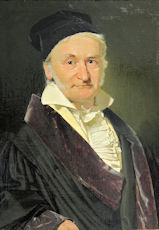# Complex NumbersCarl Friedrich Gauss - The Prince of Mathematicians

### Introduction

Complex numbers were put on a "rigorous" mathematical foundation by Carl Gauss in 1799. When a mathematician speaks of mathematical rigor, he usually means ideas are "well-defined" and the important results can be proven. We will not worry about "mathematical rigor". Our approach is going to be to develop an intuitive understanding of what complex numbers are, how to calculate with them, and how they are used in science. Remember, mathematics is the language of science, and we have no choice if understanding nature (and qubits) is our goal. Mathematical proof, while important, is secondary to this aim...
Historically, this has been a fruitful approach for math. Physicists have often created new mathematical ideas in order to solve the problems they were working on.... ideas which would seldom have occured to the mathematician in his ivory tower. The physicist creates the new mathematical idea, and the mathematician, following behind him, takes the idea and develops it into a real mathematical theory. Dirac's use of the delta function, and Schwartz's subsequent development of the theory of distributions, is perhaps the canonical example.
...next time -- more complex numbers!